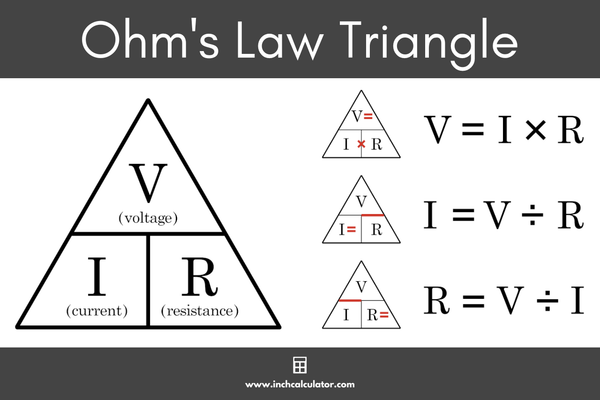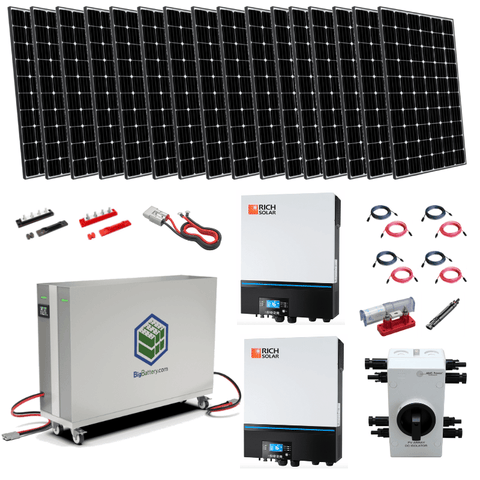Convert Volts to Watts - Knowing the How and Why - ShopSolar.com

# Convert Volts to Watts

Knowing how to convert volts to watts will make your solar or electrical calculations easier to do and understand.

Converting one unit of power to another is a matter of understanding the various laws of power.

So let’s dive in and see how we would go about converting volts to watts, and vice versa.

Before we can dive into the conversion, we should understand these units of power and discover their relationship

## The Theory Behind the Power

Knowing the definitions of the different units can help us understand where they are used and how we can obtain them.### Volts

An electrical circuit uses electrons to carry electricity, but these electrons need a form of pressure to ensure they’re always moving. This is where voltage comes into the mix.

Voltage is provided by a positively charged terminal and a negatively charged one. If we look at a battery, electrons are travelling from the negative end to the positive end.

But if you’re plugging an appliance into a wall, the voltage is supplied by your power company.

We must understand voltage as many circuits are designed to only accept a certain number of volts.

For example, if you purchase a fan and the voltage rating is higher than that of your wall socket, it could cause the fan to break and possibly smoke.

Therefore, people use appliance kWh calculators so that they know whether they have too much or too little power output running through their appliances.

### Amps

Our second point of discussion doesn’t have anything to do with the big speakers we plug our guitars into. That would be an amplifier. We want to look at Amperes (Amps).

Amps are the measure of electrical charge that flows past a given point per second.

If we take volts and amps and multiply both together, we get the flow of current as well as the pressure at which it's flowing. This takes us to our final measurement of power, watts.

### WattsWatts refers to the amount of energy consumed each time. It’s the derived unit for power in the metric system.

When you turn on the TV or charge your phone, each activity requires power, and this amount can be measured.

Time is an important part of measuring power because it is the rate of energy transfer.

But how do we use a kVA to watts calculator? How do we convert the amps we have to volts if we know the wattage? And how do we convert volts to watts?

Some can be achieved using Ohm’s Law while others can be derived from Watt’s Law

### Ohm’s Law

Ohm’s Law describes the relationship between current, resistance, and voltage. If we know the value of two of these properties, the third can be achieved by using the law.This law is typically represented using a triangle separated into 3 individual parts. The voltage is located at the top of the triangle, with the current sitting at the bottom left, and resistance on the right.

You’ll notice that on most VA to watts calculators found online, there will be a diagram and explanation of how Ohm’s law works.

The easiest way to use the triangle is to cover up the unit of power you want to work out. The remaining units are either multiplied together or divided to get the unit you’re looking for.

As an example, let’s say we want to calculate the volts of something, and we have the resistance and current.

If we cover the voltage, the current and resistance are next to each other at the bottom of the triangle. Since they’re next to each other, we need to multiply them together.

Now that we’ve understood the basics of Ohm’s Law, we can have a look at Watt’s law, which is the one we’re going to use to convert volts to watts.

### Watts Law

Watt’s law and ohm’s law are extremely like one another as they both make use of a triangle to help us solve equations of power.

This law describes the relationship between power, current, and voltage in a circuit.

Power, or watts, is located at the top of the triangle, with current and voltage being in the bottom left and right receptively.

Watts law can be used in all sorts of online calculators.

From the standard AC watts calculator for home appliances to the larger scale wattage calculations found in solar off-grid systems, you will most likely see this triangle on those sites.The same approach in Ohm’s law is used here. simply cover the unit of power you want to find the value to, and multiply or divide the remaining units.

In the case of today’s question, we wanted to figure out how to convert volts to watts.

Well, if we have a 120 V battery with a 30 A current, the watts would be 3,600 W.

## Conclusion

In most cases, converting or figuring out a unit of power is a matter of applying electrical laws.

For example, if you’re using a PV watts calculator and need to find the wattage of a solar, use one of the laws and you’re all set.

It’s a matter of seeing what you have and using that to determine what you’re missing.

Knowing how to convert volts to watts is only the one step in a long journey towards solar independence. Check out our PV Watts calculator to figure out what energy production you can expect from your location.

Did You Find Our Blog Helpful? Then Consider Checking: